Courses

# Test: CPM And PERT (Level - 3)

## 20 Questions MCQ Test Manufacturing and Industrial Engineering | Test: CPM And PERT (Level - 3)

Description
This mock test of Test: CPM And PERT (Level - 3) for Mechanical Engineering helps you for every Mechanical Engineering entrance exam. This contains 20 Multiple Choice Questions for Mechanical Engineering Test: CPM And PERT (Level - 3) (mcq) to study with solutions a complete question bank. The solved questions answers in this Test: CPM And PERT (Level - 3) quiz give you a good mix of easy questions and tough questions. Mechanical Engineering students definitely take this Test: CPM And PERT (Level - 3) exercise for a better result in the exam. You can find other Test: CPM And PERT (Level - 3) extra questions, long questions & short questions for Mechanical Engineering on EduRev as well by searching above.
QUESTION: 1

### For the project given below, the number of critical paths are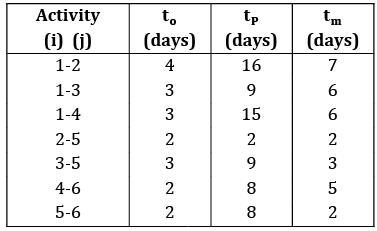Solution: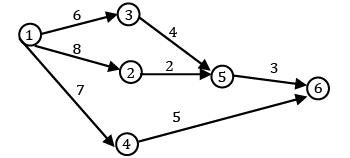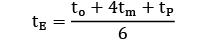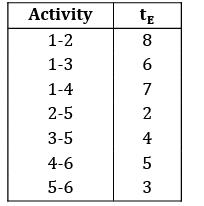Paths:

1‐ 3‐ 5‐ 6 = 6 + 4 + 3 = 13∗

1‐ 2‐ 5‐ 6 = 8 + 2 + 3 = 13∗

1‐ 4‐ 6 = 7 + 5 = 12

*Answer can only contain numeric values
QUESTION: 2

### While drawing precedence diagram for given table, no. of dummy activities required is ___________.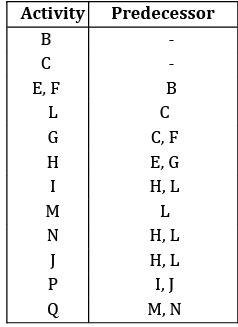Solution: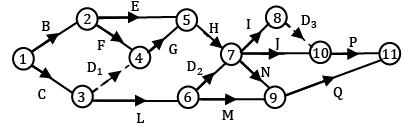No. of Dummy activities required is 3

*Answer can only contain numeric values
QUESTION: 3

### Find the completion time of the project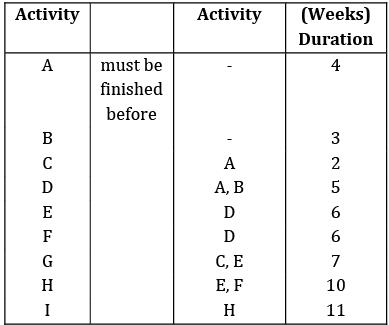(A)36(B)36

Solution: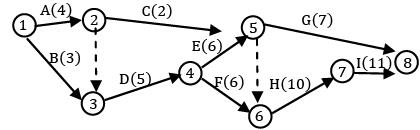Paths:

A‐ C‐ G = 4 + 2 + 7 = 13

A‐D‐E‐G = 4 + 5 + 6 + 7 = 22

A‐ D‐ F‐ H‐ I = 4 + 5 + 6 + 10 + 11 = 36∗

B‐D‐E‐G = 3 + 5 + 6 + 7 = 21

B‐ D‐ F‐ H‐ I = 3 + 5 + 6 + 10 + 11 = 35

Completion time = 36 weeks

*Answer can only contain numeric values
QUESTION: 4

A PERT network is shown below. Find the standard deviation of critical path (Upto 2 decimal places)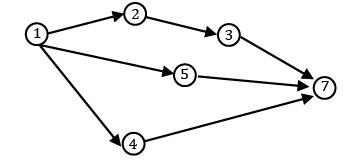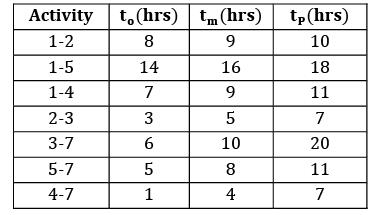Solution: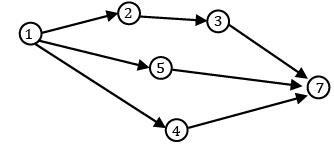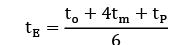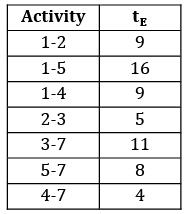Paths:

1‐ 2‐ 3‐ 7 = 9 + 5 + 11 = 25∗

1‐ 5‐ 7 = 16 + 8 = 24

1‐ 4‐ 7 = 9 + 4 = 13

standard Deviation of critical path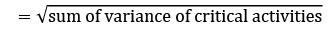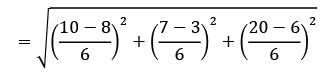= 2.45

*Answer can only contain numeric values
QUESTION: 5

A probabilistic project consist of the following activities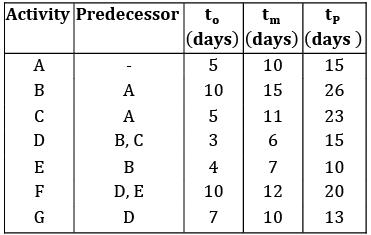Expected completion time of the project is ______________ days.

Solution: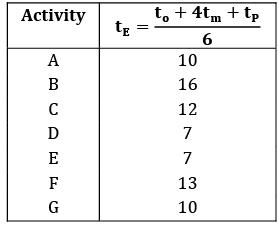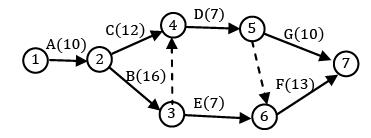Paths

A‐ C‐ D‐ G = 10 + 12 + 7 + 10 = 39

A‐ C‐ D‐ F = 10 + 12 + 7 + 13 = 42

A‐B‐D‐G = 10 + 16 + 7 + 10 = 43

A‐ B‐ E‐ F = 10 + 16 + 7 + 13 = 46

A‐ B‐ D‐ F = 10 + 16 + 7 + 13 = 46∗

QUESTION: 6

For a project following data is given: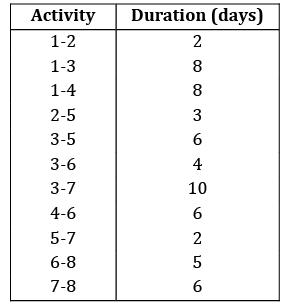The critical path and the independent float for the activity 6-8 is

Solution: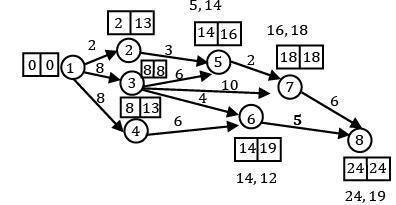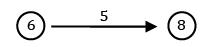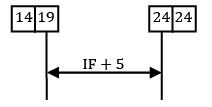IF + 5 = 24 − 19 = 5

IF = 0

QUESTION: 7

The maximum completion time of the project is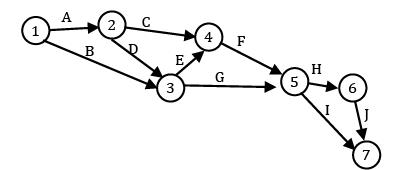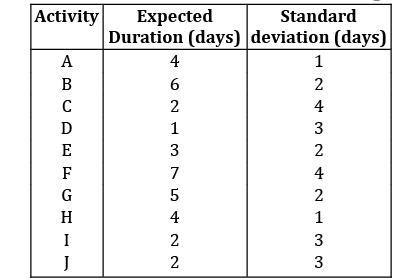Solution:

A‐C‐F‐H‐J = 4 +2 + 7+ 2+ 2 = 17

A‐C‐F‐I = 4+ 2+ 7 +2 = 15

A‐D‐E‐F‐H‐J = 4 +1 +3 + 7+ 4+ 2 = 21

A‐D‐E‐F‐I = 4+ 1+ 3+ 7 +2 = 17

A‐ D‐ G‐ H‐ J = 4 + 1 + 5 + 4 + 7 = 21

A‐ D‐ G‐ I = 4 + 1 + 5 + 2 = 12

B‐E‐F‐H‐J = 6 +3 + 7+ 4+ 2 = 22∗ = μ B‐E‐F‐I = 6+ 3+ 7 +2 = 18

B‐G‐H‐J = 6 +5 +4+2 = 17

B‐G‐I = 6+ 5+ 2 = 13

μ = 22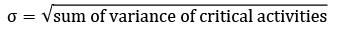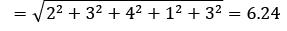Maximum completion time = μ + 3σ = 22 + 3 × 6.24

= 40.72 days

QUESTION: 8

In the network diagram shown below, the numbers adjacent to the arcs indicates the resource required to complete an activity. The resources are specified in terms of number of units. Find the critical path duration and the activity with maximum total float.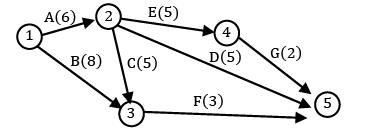Solution: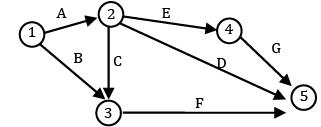A‐ E‐ G = 13

A‐ D = 11

A‐ C‐ F = 14∗

B‐ F = 11

B, D bot are having maximum total float 14, B or 14, D both are correct

QUESTION: 9

Consider the following project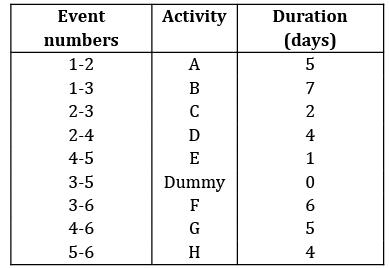Which of the following combination of activities will be having independent float as zero?

Solution: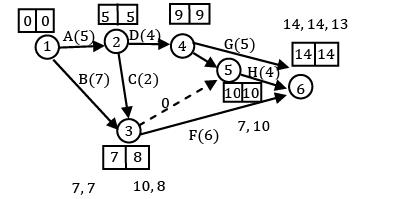The activities which are present in the critical path will have total float, free float and independent float values as zero.

QUESTION: 10

A network diagram is given below.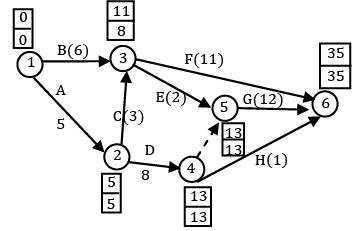Due to some technical issues if activity ‘B’ is started at time 3 and activity C is started at time 7 the EST for E and F are

Solution:

EFT )B = EST)B + duration = 3 + 6 = 9

EFT)C = 7 + 3 = 10 (max)

EST)E = (EST)F = 10

QUESTION: 11

Consider the following project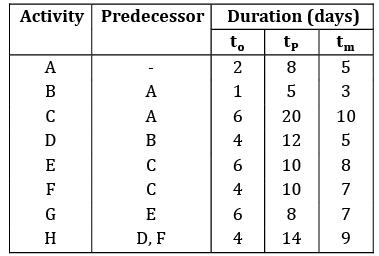The minimum completion time of the project is ___________.

Solution: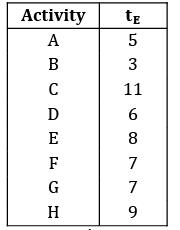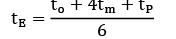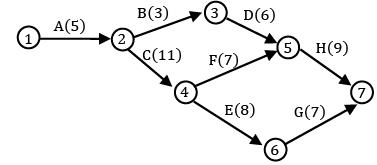Paths are:

A‐B‐D‐H = 5 + 3 + 6 + 9 = 23

A‐C‐F‐H = 5 + 11 + 7 + 9 = 32∗

A‐C‐E‐G = 5 + 11 + 8 + 7 = 31

μ = 32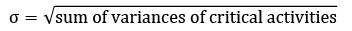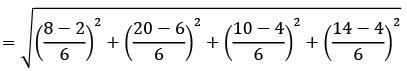σ = 3.19

Minimum completion time = μ − 3σ

= 32 − 3 × 3.19

= 22.43

QUESTION: 12

What is the critical path?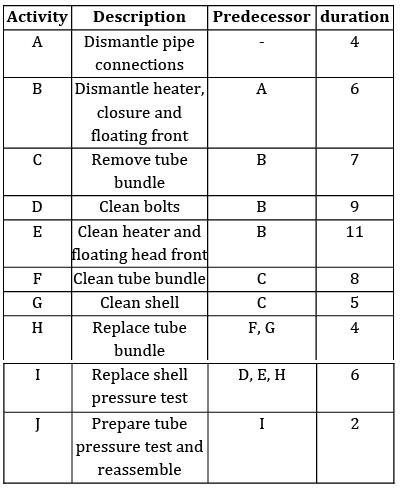Solution: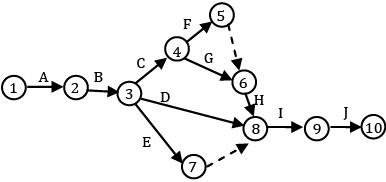Paths are:

A‐B‐C‐F‐H‐I‐J = 4 + 6 + 7 + 8 + 4 + 6 + 2 = 37∗

A‐B‐ C‐G‐H‐I‐J = 4 + 6 + 7 + 5 + 4 + 6 + 2 = 34

A‐B‐D‐I‐J = 4+ 6+ 9+ 6+ 2 = 27

A‐ B‐ E‐ I‐ J = 4 + 6 + 11 + 6 + 2 = 29

*Answer can only contain numeric values
QUESTION: 13

Expected completion time of the project is _______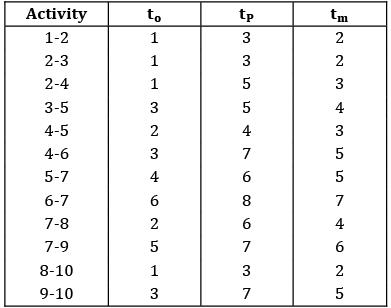Solution: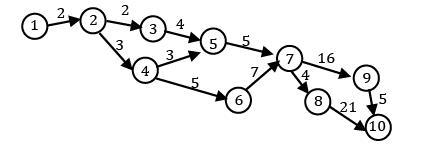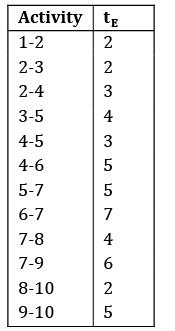1‐ 2‐ 3‐ 5‐ 7‐ 8‐ 10 = 19

1‐ 2‐ 3‐ 5‐ 7‐ 9‐ 10 = 24

1‐ 2‐ 4‐ 5‐ 7‐ 8‐ 10 = 19

1‐ 2‐ 4‐ 5‐ 7‐ 9‐ 10 = 24

1‐ 2‐ 4‐ 6‐ 7‐ 8‐ 10 = 23

1‐ 2‐ 4‐ 6‐ 7‐ 9‐ 10 = 22

*Answer can only contain numeric values
QUESTION: 14

Consider the following construction project.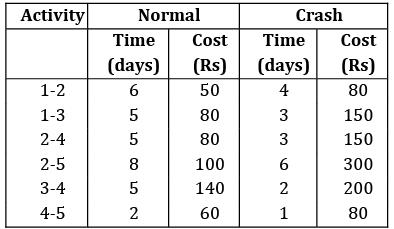If the indirect cost is Rs.20, find the optimal completion time of the project.

Solution: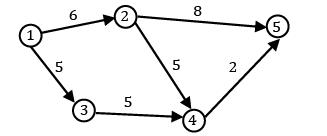Paths are:

1‐ 2‐ 5 = 6 + 8 = 14

1‐ 2‐ 4‐ 5 = 6 + 5 + 2 = 13

1‐ 3‐ 4‐ 5 = 5 + 5 + 2 = 12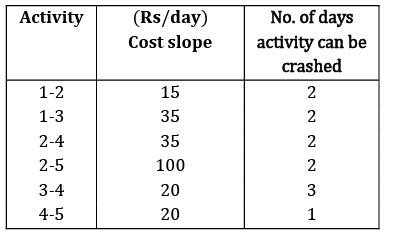i) Total cost = Direct + Indirect Direct = 50 + 80 + 80 + 100 + 140 + 60 = 510

= 510 + 20 × 14

= 790 Rs

ii) Crash ′1‐ 2′activity by 2 day TC = 510 + 15 × 2 + 20 × 12

= 780

iii) Crash 2-5, 3-4 by 1 day TC = (510 + 15 × 2 + 100 × 1 + 20 × 1)+ 20 × 11

= 880

Cost is increasing so, we can stop Optimal duration is that when the total cost is minimum Optimal completion time = 12 days

*Answer can only contain numeric values
QUESTION: 15

An R & D project has following activities to be performed. The time estimates are given in the table.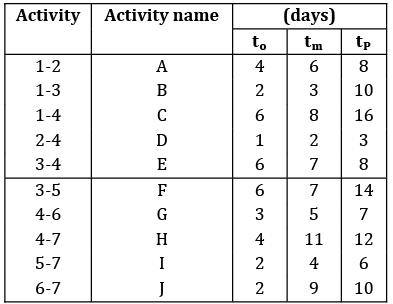The number of days required to complete the project with a probability of 50% is __________

Solution: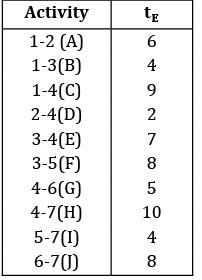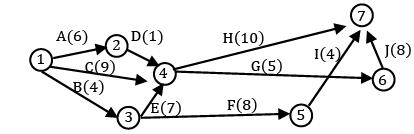A‐ D‐ H = 6 + 2 + 10 = 18

A‐D‐G‐J = 6 +2 +5 +8 = 21

C‐ H = 9 + 10 = 19

C‐G‐J = 9+ 5+ 8 = 22

B‐ E‐ H = 4 + 7 + 10 = 21

B‐E‐G‐J = 4 + 7 + 5 + 8 = 24∗

B‐F‐I = 4+ 8+ 4 = 16

Expected completion time of the project itself gives the completion time of project with 50% probability.

μ = 24

QUESTION: 16

Convert the given AON network to that of AOA network The numbers of dummy activities in the network diagram are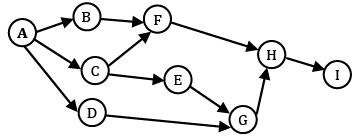Solution: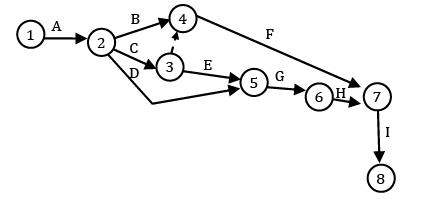No. of dummies = 1

*Answer can only contain numeric values
QUESTION: 17

A project consist of activities with the following data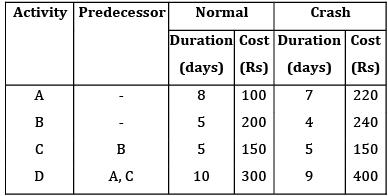The overhead cost per day is given as Rs.100. The minimum duration of the project with which minimum cost is associated ___________

Solution: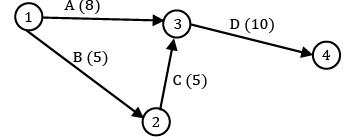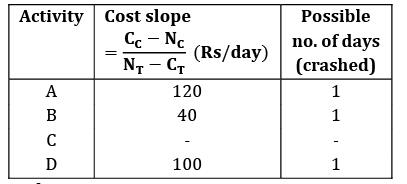Paths:

A‐ D = 8 + 10 = 18

B‐ C‐ D = 5 + 5 + 10 = 19 (critical path)

(i) Total cost = direct cost + Indirect cost

Direct cost = 100 + 200 + 150 + 300 = 750 TC = 750 + 100 × 20

= Rs. 2750

(ii) crash activity ‘B’ as it is having minimum cost slope by ‘1’ days TC

= [750 + (40 × 1) + 100 × 19

= Rs. 2690

(iii) crash activity ‘D’ by 1 day TC = (750 + 40 × 1 + 100 × 1) + 100 × 18

= 2690

No further possibility to crash minimum cost

= 2690 Duration

= 18 days

QUESTION: 18

Given that the direct fixed cost is Rs.4000. Find the optimal completion time of the project and the cost associated with it, if the overhead cost is Rs.260/day.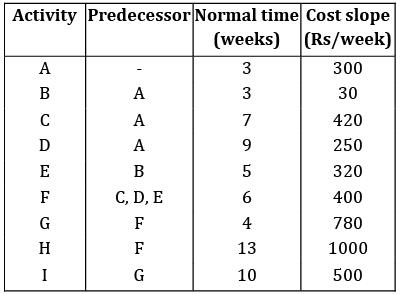Solution: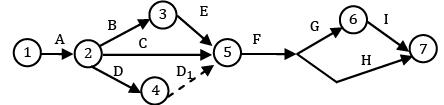Paths are:

A‐ B‐ E‐ F‐ G‐ I = 3 + 3 + 5 + 6 + 4 + 10 = 31 30

A‐ B‐ E‐ F‐ H = 3 + 3 + 5 + 6 + 10 = 27 26

A‐ C‐ F‐ G‐ I = 3 + 7 + 6 + 4 + 10 = 30

A‐C‐F‐H = 3 + 7 + 6 + 13 = 29

A‐D‐F‐G‐ I = 3 + 9 + 6 + 4 + 10 = 32∗ 31 30

A‐D‐F‐H = 3 + 9 + 6 + 13 = 31 30 29

(i) TC = 4000 + 260 × 32 = 12,320

(ii) Crash activity ‘D’ by 1 day TC = (4000 + 250 × 1) + 260 × 31

= 12,310

(iii) Crash B, D each by 1 day TC = (4000 + 250 × 1 + 30 × 1 + 250× 1) + 260 × 30

= 12,330

Optimal time = 31

Cost = 12310

*Answer can only contain numeric values
QUESTION: 19

Consider the data of a project. If the indirect cost per week is Rs.160. find the total cost after crashing two weeks?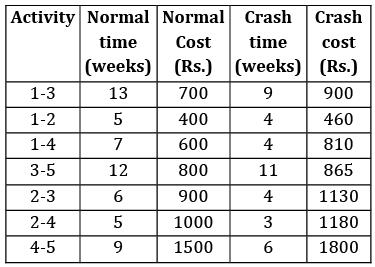(A)9670

(B)9690

Solution:

Draw a network diagram for given data and find the critical path.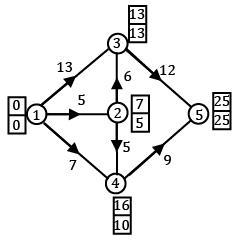Crash the critical activity 1-3 for 2 weeks.

∴ Total cost = Total normal cost +Indirect cost + slope cost − decrease in Indirect cost

= 5900 + 4000 + [50 × 2] − [160 × 2]

Total cost = 5900 + 4000 + 100 − 380 = Rs. 9680

*Answer can only contain numeric values
QUESTION: 20

A project consists of activities for which exact completion times are not known. The project consist of fair parallel paths for which the mean and variance values are given as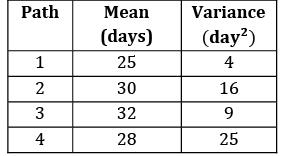What is the probability of completing the project in 29 days?

Solution:

Longer path is the path which is having maximum value of mean. It is the critical path.

Critical path duration = 32 days = μ variance = 9 = σ2

Standard deviation = σ = 3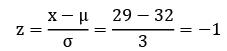z = −1

At z = −1; P = 16%

= 0.16